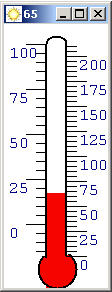Web Thermometer in C# and .NETFigure 1 - Web Thermometer

The Web Thermometer uses the same method as the WhatIsTheWeather application, GetTemperatureAndCondition, to extract the temperature from a stream on the weather.com site. The thermometer shows the temperature in both Centigrade and Fahrenheit and handles a range from 0 to 212 degrees Fahrenheit. The zip code is hard coded into the application as 10001 (NY City), so you'll need to adjust this to obtain the temperature for your area.  The temperature is updated every ten minutes with a built in timer.  The weather condition and temperature is shown as an icon in the upper left hand corner so that when the thermometer is minimized you can see the temperature on the task bar.

The Web Thermometer uses GDI+ to draw its values. Below is the code for drawing the thermometer tube.  This code draws two parallel lines and draws an arc at the top and bottom in order to create the tube.  A set of tick marks are then drawn on either side of the thermometer.  The left side shows centigrade and the right side shows Fahrenheit.  The level is calculated from the temperature and painted as a filled rectangle between the two parallel lines.

private void DrawTube(Graphics g, int temperature)
{
// Calculate the horizontal midpoint to center the thermometer in the form
int midPoint = ClientRectangle.Left + ClientRectangle.Width/2;
// Form a thick pen for outlining the tube
Pen aPen = new Pen(Brushes.Black, 2);
// draw level of fluid in the tube
g.FillRectangle(Brushes.Red, midPoint - 10, ClientRectangle.Height - ConvertTemperatureToHeight(temperature) - 30, 20,
ConvertTemperatureToHeight(temperature) + 30);
// fill arc at the bottom that holds the red fluid
g.FillPie(Brushes.Red, midPoint - 18, ClientRectangle.Height - 38, 38, 38, -55, 280);
// draw two parallel vertical lines
g.DrawLine(aPen, midPoint-10, 20, midPoint-10, ClientRectangle.Height - 30);
g.DrawLine(aPen, midPoint+10, 20, midPoint+10, ClientRectangle.Height - 32);
// calculate the height of the thermometer
int height = ClientRectangle.Height - 52;
float tickHeight = (float)height/212.0f;
// connect them with arcs
g.DrawArc(aPen, midPoint - 10, 12, 20, 15, 0, -180);
g.DrawArc(aPen, midPoint - 18, ClientRectangle.Height - 38, 38, 38, -55, 280);
// draw tics and values for Farehnheit
for (int i = 0; i < 212; i+=5)
{
// Draw tick marks every 5 degrees starting from 0 degrees Fahrenheit
g.DrawLine(Pens.Black, midPoint+10, ClientRectangle.Height - (32 + i*tickHeight), midPoint+20, ClientRectangle.Height - (32 + i*tickHeight));
// Draw text every 25 degrees starting from 0 degrees Fahrenheit
if (i % 25 == 0)
{
g.DrawLine(Pens.Black, midPoint+10, ClientRectangle.Height - (32 + i*tickHeight), midPoint+30,
ClientRectangle.Height - (32 + i*tickHeight));
g.DrawString(i.ToString(), TheFont, Brushes.Navy, midPoint + 20, ClientRectangle.Height - (32 + i*tickHeight),
new StringFormat());
}
}
// draw tics and values for Centigrade
tickHeight = (float)height/118.0f;
for (int i = 0; i <= 118; i++)
{
// Draw lines every 5 degrees starting from -18 degrees Celsius
if ( (i-18) % 5 == 0)
{
g.DrawLine(Pens.Black, midPoint-10, ClientRectangle.Height - (32 + ((
float)i)
tickHeight), midPoint-20, ClientRectangle.Height - (32 + ((
float)i)*tickHeight));
}
// Draw text every 25 degrees starting from -18 degrees Celsius
if ((i-18) % 25 == 0)
{
g.DrawLine(Pens.Black, midPoint-10, ClientRectangle.Height - (32 + ((
float)i)*tickHeight), midPoint-30,
ClientRectangle.Height - (32 + ((
float)i)*tickHeight));
g.DrawString((i-18).ToString(), TheFont, Brushes.Navy, midPoint - 50, ClientRectangle.Height - (32 + ((
float)i)*tickHeight), new StringFormat());
}
}
}

The timer in the application simply retrieves the temperature from weather.com and forces a redraw on the thermometer. Below is the event handler for the timer:

private void GetNewTemperature(object sender, System.EventArgs e)
{
GetTemperatureAndCondition(kZipCode,
ref TheTemperature, ref TheCondition);
Invalidate();
}

Improvements

It probably wouldn't be a bad idea to allow entry of the zip code in a popup menu, and then make the zip code persistent.  I was thinking it would also be nice to have a tool tip come up with a number based on the position of the mouse over the thermometer. Enjoy the weather!